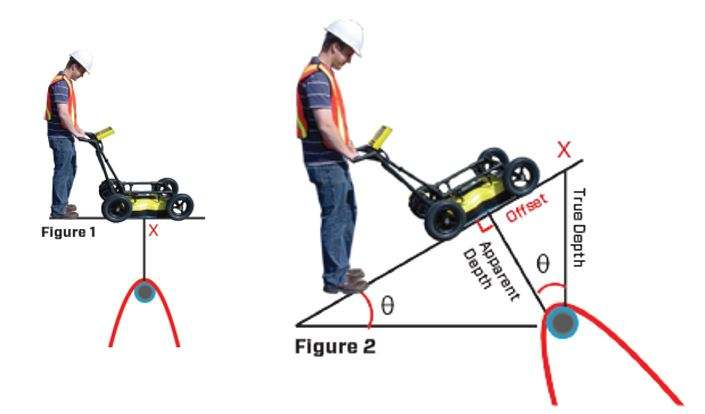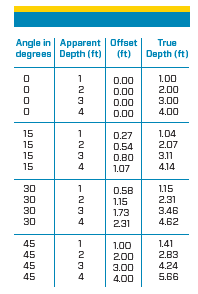Locating utility on a slopeX

# Locating utility on a slope

A

GPR traversing over objects like utility pipes and cables produce a hyperbolic time versus position response. The apex (top) of the hyperbola indicates the location where the GPR Is closest to the utility. When the surface is horizontal, the shortest path for the GPR signals to a buried object occurs when the GPR is vertically above the object (Figure 1). The utility is pinpointed by backing the GPR system up along the survey line until the screen position indicator is on top of the hyperbolic response and marking that position on the surface. Digging a vertical hole at that position will uncover the utility. The map coordinates of that position when entered into a GIS database or a CAD drawing will show the utility In the correct location on a
plan map.

When the surface Is sloping, the apex of the hyperbola, the shortest path to the utility, Is not vertical, but perpendicular to the slope surface. The position found by backing up the GPR to place the Indicator over the top of the hyperbola Is NOT the ‘correct’ location of the target; in other words, a vertical hole dug to uncover the target could miss the target If the slope Is severe.

The correct location for a vertical hole would be at the point X, shown In Figure 2. The point X is upslope from the location the GPR backup arrow would Indicate. Mathematically, the offset distance up the slope is expressed In terms of the GPR apparent depth and slope angle, 0 as:

Offset – tan(0) * Apparent Depth

The table shows the offset values for several slope angles and utility depths. The table also shows the true vertical depth of the utility; calculated by:

True Depth – Apparent Depth/Cos(θ)

The most difficult part of this calculation Is measuring the slope angle. Humans tend to over-estimate the angle of slopes, especially after climbing It. A 45 degree angle is very steep and most people would be unable to climb a slope with this angle, let alone conduct a GPR survey on it.

Notice In the table that the offset distances from low angle slopes are small enough to be Ignored in many cases.﻿

﻿